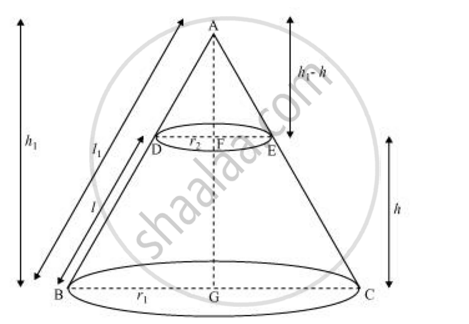Share

# Derive the Formula for the Volume of the Frustum of a Cone. - CBSE Class 10 - Mathematics

#### Question

Derive the formula for the volume of the frustum of a cone.

#### SolutionLet ABC be a cone. A frustum DECB is cut by a plane parallel to its base.

Let r1 and r2 be the radii of the ends of the frustum of the cone and h be the height of the frustum of the cone.

r_2/r_1 = (h_1-h)/h_1 = (l_1-l)/l_1

r_2/r_1 =1 -h/h_1 =1 -l/l_1

1-h/h_1= r_2/r_1

h/h_1 =1 -r_2/r_1 = (r_1-r_2)/r_1

h_1/h= r_1/(r_1-r_2)

h_1 = (r_1h)/(r_1-r_2)

Volume of frustum of cone = Volume of cone ABC − Volume of cone ADE

=1/3pir_1^2h_1 - 1/3pir_2^2(h_1-h)

=pi/3[r_1^2h_1-r_2^2(h_1-h)]

=pi/3[r_1^2((hr_1)/(r_1-r_2))-r_2^2((hr_1)/(r_1-r_2)-h)]

=pi/3[((hr_1^3)/(r_1-r_2))-r_2^2((hr_1-hr_1+hr_2)/(r_1-r_2))]

=pi/3[(hr_1^3)/(r_1-r_2)-(hr_2^3)/(r_1-r_2)]

=pi/3h[(r_1^3-r_2^3)/(r_1-r_2)]

=pi/3h[((r_1-r_2)(r_1^2+r_2^2+r_1r_2))/(r_1-r_2)]

= 1/3pih[r_1^2+r_2^2+r_1r_2]

Is there an error in this question or solution?

#### APPEARS IN

NCERT Solution for Mathematics Textbook for Class 10 (2019 to Current)
Chapter 13: Surface Areas and Volumes
Ex. 13.40 | Q: 7 | Page no. 258

#### Video TutorialsVIEW ALL 

Solution Derive the Formula for the Volume of the Frustum of a Cone. Concept: Frustum of a Cone.
S